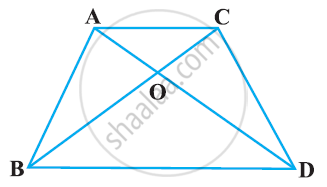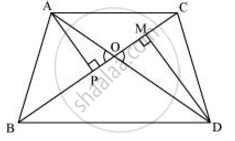# In Figure, ABC and DBC are two triangles on the same base BC. If AD intersects BC at O, show that (ar(ABC))/(ar(DBC)) = (AO)/(DO) - Mathematics

In Figure, ABC and DBC are two triangles on the same base BC. If AD intersects BC at O, show that (ar(ABC))/(ar(DBC)) = (AO)/(DO)#### Solution

Let us draw two perpendiculars AP and DM on line BC.We know that area of a triangle = 1/2 x Base x Height

:.(ar(triangleABC))/(ar(triangleDBC)) =  (1/2 BC XX AP)/(1/2BCxxDM)) = (AP)/(DM)

In ΔAPO and ΔDMO,

∠APO = ∠DMO (Each = 90°)

∠AOP = ∠DOM (Vertically opposite angles)

∴ ΔAPO ∼ ΔDMO (By AA similarity criterion)

:. (AP)/(DM) = (AO)/(DO)

=> (ar(triangleABC))/(ar(triangleDBC))=(AO)/(DO)

Concept: Areas of Similar Triangles
Is there an error in this question or solution?

#### APPEARS IN

NCERT Class 10 Maths
Chapter 6 Triangles
Exercise 6.4 | Q 3 | Page 144
RD Sharma Class 10 Maths
Chapter 7 Triangles
Exercise 7.6 | Q 19 | Page 96